Practice the AP 10th Class Physical Science Bits with Answers Chapter 5 Human Eye and Colourful World on a regular basis so that you can attempt exams with utmost confidence.

## AP State Syllabus 10th Class Physical Science Bits 5th Lesson Human Eye and Colourful World with Answers

CONCEPT – I : (Structure Of human Eye)

Question 1.
The value of “least distance of distinct vision” for young adult human being is ………………
A) 25 cm
B) 5 cm
C) 50 cm
D) infinity
A) 25 cm

Question 2.
The value of “least distance of distinct vision” at young age i.e below 10 years ……………….
A) 25 cm
B) 0 cm
C) infinity
D) 7 to 8 cm
D) 7 to 8 cmQuestion 3.
The value of “least distance of distinct vision” at old age ………………..
A) 25 cm
B) infinity
C) 7 to 8 cm
D) 1 to 2 m
D) 1 to 2 m

Question 4.
Statement X : The value of least distance of distinct vision varies from person to person and with age.
Statement Y : At young age (i.e below 10 years) the value of least distance of distinct vision is as close as 7to 8 cm due to the muscles around the eye are strong and flexible and can bear more strain.
A) Both X and Y are true
B) Both X and Y are false
C) X is true, Y is false
D) X is false, Y is true
A) Both X and Y are true

Question 5.
Statement X : The value of least distance of distinct vision does not change with age.
Statement Y : In old age the muscles cannot sustain more strain hence the value of least distance of distinct vision shifts to a larger value.
A) Both X and Y are true
B) Both X and Y are false
C) X is true, Y is false
D) X is false, Y is true
D) X is false, Y is true

Question 6.
The range of vision for a young adult with normal vision ……………………..
A) 0 to 25 cm
B) 25 cm to 200 cm
C) 25 cm to infinity
D) zero to infinity
C) 25 cm to infinity

Question 7.
The angle of vision for a healthy human being is……………….
A) 60°
B) 70°
C) 80°
D) 180°
A) 60°

Question 8.
The maximum angle at which we are able to see the whole object is called ……………….
A) persistance of vision
B) angle of vision
C) least distance of distinct vision
D) none
B) angle of vision

Question 9.
Statement X : The value of least distance of distinct vision varies from person to person and with age.
Statement Y : The angle of vision varies from person to person and with age.
A) Both X and Y are true
B) Both X and Y are false
C) X is true, Y is false
D) X is false, Y is true
A) Both X and Y are trueQuestion 10.
If the angle of vision is more than 60° then you can see ………………….
A) the whole object
B) only the part of the object
C) both A and B
D) none
B) only the part of the object

Question 11.
The minimum distance from the eye at which the eye can see the objects clearly and distinctly without any strain is called …………………
A) the maximum distance of distinct vision
B) the average distance of distinct vision
C) the least distance of distinct vision
D) none
C) the least distance of distinct vision

Question 12.
The far point of human eye with normal vision lies at ………………..
A) 25 cm
B) 20 cm
C) 0 cm
D) infinity
D) infinity

Question 13.
Name the front part of the eye.
A) cornea
B) iris
C) aqueous humour
D) pupil
A) cornea

Question 14.
Name the coloured part that we see in an eye.
A) cornea
B) iris
C) ciliary muscles
D) pupil
B) iris

Question 15.
Name the part of the eye which controls the amount of light entering the eye.
A) cornea
B) iris
C) pupil
D) retina
B) iris

Question 16.
Name the liquid between the cornea and eye lens of the eye.
A) pupil
B) vitreous humour
C) aqueous humour
D) iris
A) pupil

Question 17.
Name the part of the eye which is a hole in the middle of the iris …………………
A) eye lens
B) retina
C) iris
D) pupil
C) irisQuestion 18.
Name the part of the eye that appears black.
A) eye lens
B) retina
C) iris
D) pupil
D) pupil

Question 19.
The size of the pupil in low light condition …………………
A) increases
B) decreases
C) remains same
D) none
D) none

Question 20.
The size of the pupil in the case of bright (or) excess light condition ………………….
A) increases
B) decreases
C) remains same
D) none
A) increases

Question 21.
The size of the pupil …………….. when you entered into a cinema hall.
A) increases
B) decreases
C) remains same
D) none
B) decreases

Question 22.
…………….. lens is present in the human eye.
A) Concave
B) Convex
C) Both
D) None
A) Concave

Question 23.
The human eye forms the image of an object at its ……………………
A) cornea
B) iris
C) retina
D) eyeball
C) retina

Question 24.
The distance between the eye lens and retina is …………………..
A) 2.25 cm
B) 2.27 cm
C) 2.5 cm
D) 5 cm
C) 2.5 cm

Question 25.
Statement X : The image distance is fixed which is formed by eye lens.
Statement Y : The image formed on our retina is upside down.
A) Both statements are true
B) Both statements are false
C) X is true, Y is false
D) Y is false, Y is true
A) Both statements are trueQuestion 26.
The change in focal length of an eye lens is caused by the action of the ………………..
A) pupil
B) retina
C) ciliary muscles
D) iris
C) ciliary muscles

Question 27.
When the eye is focused on a closer object the focal length of the eye lens ………………..
A) increases
B) decreases
C) remains same
D) none
B) decreases

Question 28.
When the eye is focused on a distant object the focal length of the eye lens …………………….
A) increases
B) decreases
C) remains same
D) none
A) increases

Question 29.
The term “accommodation” as applied to the eye, refers to its ability to …………………..
A) control the light intensity falling on the retina.
B) vary the distance between eye lens and retina.
G) erect the inverted image on the retina.
D) change the focal length of the lens.
D) change the focal length of the lens.

Question 30.
Statement X : The.focal length of the eye lens has maximum when the eye is focused on a distant object to focus the image.
Statement Y : The focal length of the eye lens has maximum when the eye is focused ‘ on a closer object to focus the image.
A) Both statements are true
B) Both statements are false
C) X is true, Y is false
D) X is false, Y is.true
A) Both statements are true

Question 31.
Statement X : When the eye is focused on a closer object the ciliary muscles are strained and focal length of the eye lens increases.
Statement Y : When the eye is focused on a distant object the ciliary muscles are relaxed and focal length of the eye lens|decreases.
A) Both statements are true
B) Both statements are false
C) X is true, Y is false
D) X is false, Y is true
B) Both statements are false

Question 32.
Refraction of light in the eye occurs at …………………..
A) only the lens
B) only the cornea
C) both the cornea and lens
D) none
A) only the lensQuestion 33.
Statement X : The eye lens forms a real and inverted image of an object on the retina.
Statement Y : The eye lens forms a virtual and erect image of an object on the retina.
A) Both statements are true
B) Both statements are false
C) X is true, Y is false
D) X is false, Y is true
C) X is true, Y is false

Question 34.
The range of focal length of an eye lens is…………………..
A) 0 to ∝
B) 2.25 to 2.50 cm
C) 2.27 to 2.50 cm
D) 0 to 25
C) 2.27 to 2.50 cm

Question 35.
The value of focal length of the eye lens when an object is at infinity is ………………………
A) 2.50 cm
B) 2.25 cm
C) 2.27 cm
D) can’t find
A) 2.50 cm

Question 36.
The value of focal length of the eye lens when an object is placed at a distance of least distance of distinct vision is …………………….
A) 2.50 cm
B) 2.25 cm
C) 2.27 cm
D) 25cm
C) 2.27 cm

Question 37.
The value of focal length of the eye lens when the object is between infinity and the point of least distance of distinct vision is ……………………
A) greater than 2.5 cm
B) less than 2.27 cm
C) greater than 25 cm
D) between 2.27 cm to 2.50 cm
D) between 2.27 cm to 2.50 cm

Question 38.
The ability of eye – lens to change its focal length is called…………………..
A) power of lens
B) accommodation of lens
C) co-ordination of lens
D) none
B) accommodation of lens

Question 39.
The part of the eye sensitive to light is ……………………….
A) retina
B) pupil
C) ciliary muscles
D) cornea
A) retinaQuestion 40.
The cells preseritiri the retina of an eye which identify the colour of an object are ……………………
A) rods
B) cones
C) both rods arid cones
D) none
B) cones

Question 41.
The cells present in the retina of an eye which are sensitive to intensity of light are……………….
A) rods
B) cones
C) both rods and cones
D) none
A) rods

Question 42.
The light signals are transmitted to the brain through ……………………….
A) eye lens
B) pupil
C) retina
D) optic nerves
D) optic nerves

Question 43.
The function of the rods of the retina is to ……………………
A) adjust focal length of the eye lens
B) enable pupil to expand or contract to receive light
C) identify the intensity of light
D) identify the colour of light
C) identify the intensity of light

Question 44.
The maximum and minimum focal lengths of the eye lens respectively are ………………….
A) 2 cm, 1cm
B) 2.25 cm, 1.5 cm
C) 2.5 cm, 2.27 cm
D) 25 cm, 2.27 cm
C) 2.5 cm, 2.27 cm

Question 45.
Select the incorrect statement of the following.
A) The least distance of distinct vision at below 10 years age is 7- 8 cm
B) The least distance of distinct vision in old age is 1 m or even more
C) If angle of vision is above 60° then we can see only the part of the object
D) Angle of vision and least distance of distinct vision are same for all persons
D) Angle of vision and least distance of distinct vision are same for all persons

Question 46.
Which part of human eye is called “Variable aperture”?
A) retina
B) cornea
C) pupil
D) lens
C) pupil

Question 47.
For any position of an object in front of the human eye the image distance is fixed at ………………………
A) 1 cm
B) 1.5 cm
C) 2.5 cm
D) 0.25 cm
C) 2.5 cmQuestion 48.
Which of the following changes takes place when you walk out of bright sunshine into a poorly light room ?
A) The pupil becomes larger
B) The lens becomes thicker
C) The ciliary muscles relax
D) The pupil becomes smaller
D) The pupil becomes smaller

Question 49.
When objects at different distances are seen by the eye one of the following remains constant.
A) focal length of the human eye lens
B) object distance from eye lens
C) the radii of curvature of eye lens
D) image distance from eye lens
D) image distance from eye lens

Question 50.
Which part of the human eye contains “rods” and “cones” to receive the light signals?
A) cornea
B) iris
C) pupil
D) retina
D) retina
Question 51.
For any position of the object in front of the human eye the image distance is ………………
A) 25 cm
B) 5 cm
C) 2.5 cm
D) 1 cm
C) 2.5 cm

CONCEPT – II : Defects Of Visions – Their Corrections

Question 52.
Which of the following is common defect of vision ?
A) Myopia
B) Hypermetropia
C) Presbyopia
D) All
D) All

Question 53.
Some people can not see ob j ects at long distances but can see nearby objects clearly. This type of defect in vision is called ………………..
A) myopia
B) near sightedness
C) both A and B
D) hypermetropia
C) both A and B

Question 54.
Some people can not see ob j ects at near distances but can see distant objects clearly. This type of defect in vision is called………………..
A) hypermetropia
B) long sightedness
C) both A and B
D) myopia
C) both A and B

Question 55.
The maximum focal length of the myopia eye is …………………
A) 2.5 cm
B) less than 2.5 cm
C) more than 2.5 cm
D) 22.7 cm
B) less than 2.5 cmQuestion 56.
The minimum focal length of the hypermetropic eye is ……………….
A) 2.27cm
B) lessthan 2.27 cm
C) greaterthan 2.27 cm
D) 2.5 cm
C) greaterthan 2.27 cm

Question 57.
The point of maximum distance at which the eye lens can form an image on the retina is called
A) far point
B) near point
C) focal point
D) pupil
A) far point

Question 58.
For normal vision the far point is ……………….
A) 25 cm
B) 2.5 cm
C) 0
D) at infinity
D) at infinity

Question 59.
For myopic eye the far point lies ………………………
A) at 25 cm
B) between 25 cm and infinity
C) lessthan 25 cm
D) at infinity
B) between 25 cm and infinity

Question 60.
For hypermetropic eye the far point lies …………………..
A) at 25 cm
B) at lessthan 25 cm
C) at infinity
D) at zero
C) at infinity

Question 61.
The defect in which people can not see objects beyond far point is called …………………
A) myopia
B) hypermetropia
C) presbyopia
D) none
A) myopia

Question 62.
The point of minimum distance at which the eye lens cap form an image on the retina is called ……………………..
A) far point
B) near point
C) focal point
D) pupil
B) near pointQuestion 63.
The defect in which people can not see objects between near point and point of least distance of distinct vision is called …………………….
A) myopia
B) hypermetropia
C) presbyopia
D) none
B) hypermetropia

Question 64.
Statement X : In myopia people can not see objects beyond far point.
Statement Y : In hypermetropia people can see objects beyond near point.
A) Both statements are true
B) Both statements are false
C) X is true, Y is false
D) X is false, Y is true
A) Both statements are true

Question 65.
For a myopic eye the image is formed ………………. when the object is placed beyond the far point.
A) at retina
B) before the retina
C) beyond the retina
D) none
B) before the retina

Question 66.
For a hypermetropic eye the image is formed……………., when the object is placed between near point and point of least distance of distinct vision.
A) at retina
B) before the retina
C) beyond the retina
D) none
C) beyond the retina

Question 67.
Myopia can be corrected by using …………………….
A) concave lens
B) convex lens
C) bi focal lens
D) concave mirror
A) concave lens

Question 68.
Hypermetropia can be corrected by using ………………….
A) concave lens
B) convex lens
C) bi focal lens
D) convex mirror
B) convex lensQuestion 69.
Which of the following ray diagram is correct for myopia defect ?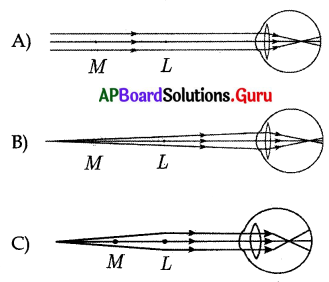D) all
D) all

Question 70.
Which of the following ray diagram is correct for hypermetropia defect.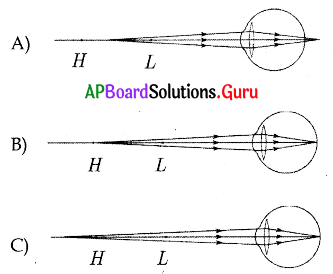D) All
D) All

Question 71.
The focal length of the lens to be used to correct myopia when the far point distance is “d” cm.
A) f = 25d/ (d – 25)
B) f = -d
C) f = (d – 25)/ 25d
D) f = $$\frac{1}{\mathrm{~d}}$$
B) f = -d

Question 72.
The focal length of the lens to be used to correct hypermetropia when the near point distance is “d” cm.
A) f = 25d/ (d – 25)
B) f = -d
C) f = (d-25)/ 25d
D) f = $$\frac{1}{\mathrm{~d}}$$
A) f = 25d/ (d – 25)

Question 73.
The ability of accommodation of the eye usually decreases with ageing. This type of defect in vision is called ………………….
A) myopia
B) hypermetropia
C) cataract
D) presbyopia
D) presbyopia

Question 74.
A person suffering from myopia and hypermetropia uses ………………. lens.
A) convex
B) concave
C) bifocal
D) cylindrical
C) bifocal

Question 75.
Statement X : Bifocal lens has concave lens at its upper portion and convex lens ‘at its lower portion.
Statement Y : Bifocal lens has convex lens at its upper portion and concave lens at its lower portion.
A) Both statements are true
B) Both statements are false
C) X is true, Y is false
D) X is false, Y is true
C) X is true, Y is false

Question 76.
Presbyopia can be corrected by using ………………… lens.
A) convex
B) concave
C) bifocal
D) cylindrical
C) bifocalQuestion 77.
The degree of convergence or divergence of light rays that can be achieved by a lens is called …………………..
A) accommodation
B) power
C) focal length
D) none
C) focal length

Question 78.
The reciprocal of focal length of a lens is called ……………………….
A) accommodation
B) power
C) focal length
B) power

Question 79.
The unit of power is ………………………
A) diopter
B) meter
C) centimetre
D) 1/diopter
A) diopter

Question 80.
See figure, parallel rays of light fall on eye and they converge before the retina. It represents a certain defect in the eye. To correct it …………….. lens is used.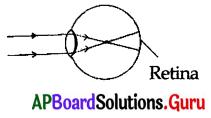A) bi convex
B) biconcave
C) convex (or) cbncave
D) concavo – convex
B) biconcave

Question 81.
Doctor tested the eyes of Raj Kumar and identified that he had hypermetropia. The distance of near point is 50 cm. The power of the lens suggested by the doctor to him is ……………………..
A) -2D
B) + 1D
C) – 1D
D) + 2D
D) + 2D

Question 82.
In case of eye defect called “myopia” image is formed ………………………
A) after retina
B) before retina
C) on retina
D) none
B) before retina

Question 83.
If a person uses a lens of -2.5 D power, then the focal length of the lens is ……………………
A) 40 cm
B) 50 cm
C) -40 cm
D) -50 cm
C) -40 cm

Question 84.
Find the focal length of the lens if the power of the lens is – 2D
A) 49 cm
B) 50 cm
C) 52.5 cm
D) 55 cm
B) 50 cmQuestion 85.
A doctor advised to use 4D lens. The focal length of the lens is ……………………..
A) 25cm
B) 400cm
C) 4 cm
D) 40 cm
A) 25cm

Question 86.
Which of the following statements is correct ?
A) A person with hypermetropia can see near by objects clearly
B) A person with myopia can see near by objects clearly
C) A person with hypermetropia can not see distant objects clearly
D) A person with myopia can see distant objects clearly
B) A person with myopia can see near by objects clearly

Question 87.
If far point of a long sighted person is ‘x’. The focal length of the lens he has to use is …………………..
A) $$\frac{25 x}{x-25}$$
B) $$\frac{25 x}{x+25}$$
C) $$\frac{x-25}{25 x}$$
D) $$\frac{x+25}{25 x}$$
A) $$\frac{25 x}{x-25}$$

Question 88.
The minimum focal length of eye lens for the person of hypermetropia is greater than …………………
A) 2.27 cm
B) 2.5 cm
C) 2.5 m
D) 2.27 m
A) 2.27 cm

Question 89.
A doctor has advised to use 2.5D lens. The focal length of the lens is …………………..
A) 40 cm
B) 250 cm
C) 2.5 cm
D) 50 cm
A) 40 cm

Question 90.
Ramu can read a book perfectly well but cannot read the writing on black board unless he sits on the front row in class. Name the defect he has …………………..
A) myopia
B) hypermetropia
C) presbyopia
D) astigmatism
A) myopia

Question 91.
A man can read the number of a distant bus clearly but he finds difficulty in reading a book. The defect of vision he has is …………………….
A) myopia
B) hypermetropia
C) presbyopia
D) astigmatism
B) hypermetropiaQuestion 92.
A student sitting in the last row of the class room is not able to read clearly the writing on the black board. Name the defect he is suffering from.
A) myopia
B) hypermetropia
C) presbyopia
D) astigmatism
A) myopia

Question 93.
A doctor has advised to use + 1D and + 1.5D lens for his left eye and right eye respectively of a patient. What type of defect he has …………………..
A) short – sightedness
B) long-sightedness
C) cataract
D) presbyopia
B) long-sightedness

Question 94.
A student sitting on the last bench in the class cannot read the writing on the black board clearly but he can read the book lying on his desk clearly. Which of the following statements is correct about the student ?
A) The near point of his eyes has receded away
B) The near point of his eyes has, come closer to him
C) The far point of his eyes has receded away
D) The far point of his eyes has come closer to him
D) The far point of his eyes has come closer to him

Question 95.
Far point of a person is 5 m. In order to have normal vision what kind of spectacles should he use ?
A) Concave lens with focal length 5 m
B) Concave lens with focal length 10 m
C) Convex lens with focal length 5 m
D) Convex lens with focal length 2.5 m
A) Concave lens with focal length 5 m

CONCEPT – III : Dispersion and scattering of light

Question 96.
A transparent medium separated from – the surrounding medium by atleast two plane surfaces which are inclined at a . certain angle in such a way that light incident on one of the plane surfaces emerges from the other plane surface is called ……………………..
A) a rectangular glass slab
B) a prism
C) a mirror
D) a lens
B) a prism

Question 97.
Which of the following statements is false?
A) The angle between the two rectangular plane surfaces of a prism is called the angle of the prism.
B) The angle between the emergent ray and normal is called the angle of emergence.
C) The angle between the emergent ray and incident ray is called angle of deviation.
D) The angle between the incident ray and refracted ray is called angle of deviation.
D) The angle between the incident ray and refracted ray is called angle of deviation.

Question 98.
Identify correctly marked angles from the figure.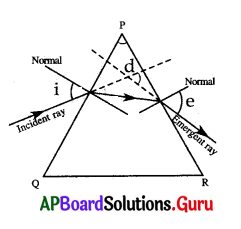A) only i
B) i and e
C) i, e and d
D) none
C) i, e and dQuestion 99.
A ray of light falls on one of the lateral surface of an equilateral prism placed on the horizontal surface of a table as shown in figure. For minimum deviation of ray which of the following is true?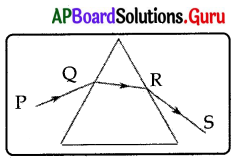A) PQ is horizontal
B) QR is horizontal
C) RS is horizontal
D) Either PQ or RS is horizontal
B) QR is horizontal

Question 100.
A ray of light incident with 45° on one of the lateral surface of an equilateral prism placed on the horizontal surface of a table. Find the minimum deviation produced by a prism.
A) 45°
B) 30°
C) 60°
D) 90°
B) 30°
Question 101.
Find angle of a glass prism from the graph.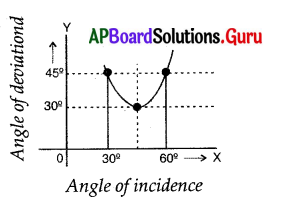A) 30°
B) 45°
C) 60°
D) 90°
C) 60°

Question 102.
Statement X : For minimum angle of deviation the angle of incidence is equal to angle of emergence.
Statement Y : For minimum angle of deviation the incidentray is parallel to the emergent ray.
A) Both statements are true
B) Both statements are false
C) X is true, Y is false
D) X is false, Y is true
A) Both statements are true

Question 103.
The relation among angle of incidence (i1) angle of emergence (i1) angle of deviation (d) and angle of prism (A)
A) A + i1 = d + i2
B) A – d = i1 + i2
C) A + d = i1 + i2
D) A + d = i1 – i2
C) A + d = i1 + i2

Question 104.
The formula for the refractive index of the prism …………………..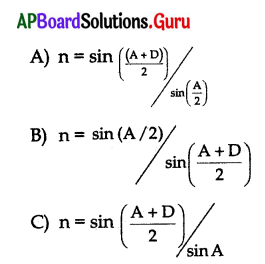D) n = sin (A + D) / sin A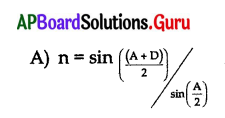Question 105.
A prism with an angle A = 60° produces an angle of minimum deviation of 30°. Find the refractive index of the prism.
A) √3
B) $$\frac{1}{\sqrt{2}}$$
C) √2
D) 2
C) √2

Question 106.
The value of angle of deviation produced by a prism …………………….
A) increases with increase in the value of angle of incidence
B) increases with increase in the value of wavelength of incident
C) decreases first and then increases with increase in the angle of incidence
D) increases first and then decreases with increase in the angle of incidence
C) decreases first and then increases with increase in the angle of incidenceQuestion 107.
The splitting of white light into different colours is called…………
A) dispersion
B) scattering
C) refraction
D) total internal reflection
A) dispersion

Question 108.
The colour of white light which suffers the maximum bending on passing through a glass prism is …………………….
A) red
B) yellow
C) green
D) violet
D) violet

Question 109.
The colour of white light which suffers the minimum refraction on passing through a glass prism is …………………
A) red
B) yellow
C) orange
D) violet
A) red

Question 110.
In the spectrum of white light the two colours appearing at the ends are ………………
A) red, violet
B) green, yellow
C) blue, red
D) red, orange
A) red, violet

Question 111.
Statement X : The speed of light is constant in vacuum for all colours.
Statement Y : The speed of light is constant in medium for all colours.
A) Both statements are true
B) Both statements are false
C) X is true, Y is false
D) X is false, Y is true
C) X is true, Y is false

Question 112.
Statement X : The refractive index of medium depends on wavelength of light.
Statement Y : The refractive index decreases with an increase in wavelength.
A) Both statements are true
B) Both statements are false
C) X is true, Y is false
D) X is false, Y is true
A) Both statements are true

Question 113.
Which of the following colour of white light has least Wavelength ?
A) Red
B) Violet
C) Indigo
D) Blue
B) VioletQuestion 114.
Which of the following colour of white light has maximum wavelength ?
A) Green
B) Orange
C) Violet
D) Red
D) Red

Question 115.
The relation between wavelength and deviation is ……………………
A) wavelength ∝ deviation
B) wavelength ∝ $$\frac{1}{\text { deviation }}$$
C) wavelength = deviation
D) none
B) wavelength ∝ $$\frac{1}{\text { deviation }}$$

Question 116.
The relation between the speed of a wave (υ), wavelength and (λ) frequency
(f) is ……………………
A) f = υ λ
B) υ = f λ
C) λ = f υ
D) λ = $$\frac{f}{v}$$
B) υ = f λ

Question 117.
The number of waves leaving the source per second is called …………………..
A) frequency
B) wavelength
C) speed of the wave
D) none
A) frequency

Question 118.
Which of the following does not change due to refraction ?
A) Wavelength
B) Speed of the wave
C) Frequency
D) None
C) Frequency

Question 119.
Statement X : Speed of the wave increases with increase in , wavelength of light.
Statement Y : Frequency does not change due to refraction.
A) Both statements are true
B) Both statements are false
C) X is true, Y is false
D) X is false, Y is true
A) Both statements are true

Question 120.
Bright rainbow can be observed when the angle between incoming and outgoing light ray in a rain drop is …………………
A) 42°
B) 0° to 40°
C) 40°
D) 0° to 42°
D) 0° to 42°Question 121.
The red colour in a rainbow will be seen when the angle between a beam of sunlight and light sent back by a raindrop ………………….
A) 42°
B) 0° to 40°
C) 40°
D) 0° to 42°
A) 42°

Question 122.
The violet colour in a rainbow will be seen when the angle between a beam of sunlight and light sent back by a raindrop ……………….
A) 42°
B) 40°
C) 0° to 42°
D) 0° to 40°
B) 40°

Question 123.
If we look at the raindrops dispersing sunlight at an angle between 40° and 42° ………………..
A) we can observe only violet
B) we can observe only red
C) we can observe VIBGYOR
D) we can observe VIBG only
C) we can observe VIBGYOR

Question 124.
The light dispersed by the rain drops appears as a bow because ……………………
A) all drops that disperse the light towards us lie in the shape of bow
B) all drops that disperse the light towards us lie in the shape of cone with our eye as tip
C) all drops that disperse the light towards us lie in the shape of circle.
D) none
B) all drops that disperse the light towards us lie in the shape of cone with our eye as tip

Question 125.
Rainbow contains ………………………
A) red colour on the outermost layer
B) violet colour on the outermost layer
C) red colour on the innermost layer
D) yellow colour on the outermost layer
A) red colour on the outermost layer

Question 126.
Statement X : A rainbow is always formed in a direction opposite to that of the sun.
Statement Y : A rainbow is produced by the dispersion of white sunlight by rain drop in the atmosphere.
A) Both statement are true
B) Both statement are false
C) X is true, Y is false
D) X is false, Y is true
A) Both statement are true

Question 127.
Which one of the following phenomena of light are involved in the formation of a rainbow?
A) refraction, dispersion
B) refraction, dispersion and total internal reflection
C) reflection, refraction and dispersion
D) dispersion, scattering and total internal reflection
B) refraction, dispersion and total internal reflectionQuestion 128.
In prism experiment the i – d curve looks like ………………………
A) straight line
B) circle
C) parabola
D) elipse
C) parabola

Question 129.
The splitting of white light into different colours is called …………………..
A) dispersion
B) scattering
C) refraction
D) reflection
A) dispersion

Question 130.
Light is a/an ……………………….
A) longitudinal wave
B) stationary wave
C) electromagnetic wave
D) none
C) electromagnetic wave

Question 131.
What happens to refractive index of a medium when wavelength of incident light ray increases?
A) Decreases
B) Increases
C) Becomes zero
D) Becomes infinity
A) Decreases

Question 132.
The reason for low angle of deviation for red colour in dispersion of light is due to its …………………
A) low refractive index
B) high refractive index
C) low wavelength
D) frequency
A) low refractive index

Question 133.
The formation of rainbow in the sky is due to the dispersion of sunlight by ………………….
A) clouds
B) water droplets
C) air molecules
D) water in the sea
B) water droplets

Question 134.
a) Red colour has the shortest wavelength.
b) Formation of rainbow is an example for dispersion.
A) only (a) is true
B) only (b) is true
C) (a) is false; (b) is true
D) both (a) and (b) are false
C) (a) is false; (b) is true

Question 135.
The process of re-emission of absorbed light in all directions with different intensities by atoms or molecules is called………………..
A) scattering of light
B) dispersion of light
C) refraction of light
D) reflection of light
A) scattering of lightQuestion 136.
A small particle will scatter light of ……………. wavelength better.
A) large
B) shorter
C) medium
D) none
B) shorter

Question 137.
Statement X : The intensity of scattered light varies with angle of scattering.
Statement Y : The intensity of scattered light is maximum at 90° of angle of scattering.
A) Both statements are true
B) Both statements are false
C) X is true, Y is false
D) X is false, Y is true
A) Both statements are true

Question 138.
The blue colour of the sky is due to ……………………
A) refraction of light
B) dispersion of light
C) diffraction of light
D) scattering of light
D) scattering of light

Question 139.
The sky appears blue because some of the blue component of sunlight is scattered by
A) gas molecules present in air
B) dust particles present in air
C) water droplets suspended in air
D) soot particles present in air
A) gas molecules present in air

Question 140.
Which of the following molecules are responsible for the sky appearing blue
A) N2
B) O2
C) H2O
D) Both A and B
D) Both A and B

Question 141.
The scientific work of C.V. Raman is on
A) dispersion of light
B) total internal reflection of light
C) defects of vision
D) scattering of light
D) scattering of light

Question 142.
The red colour of the sun at the time of sunrise and sunset is because
A) red colour is least scattered
B) blue colour is least scattered
C) red colour is most scattered
D) blue colour is most scattered
A) red colour is least scattered

Question 143.
Assertion (A) : Blue colour of the sky is due to scattering of light.
Reason (R) : Blue colour has shortest wavelength among all colours of white light.
A) Both A and R are true
B) Both A and R are false
C) A is true but R is false
D) A is false but R is true
C) A is true but R is falseQuestion 144.
Assertion (A) : The refractive index of a prism depends only on the kind of a glass which is made of and the colour of light.
Reason (R) : The refractive index of a prism depends on the refracting angle of a prism and the angle of minimum deviation.
A) Both A and R are true
B) Both A and R are false
C) A is true but R is false
D) A is false but R is true